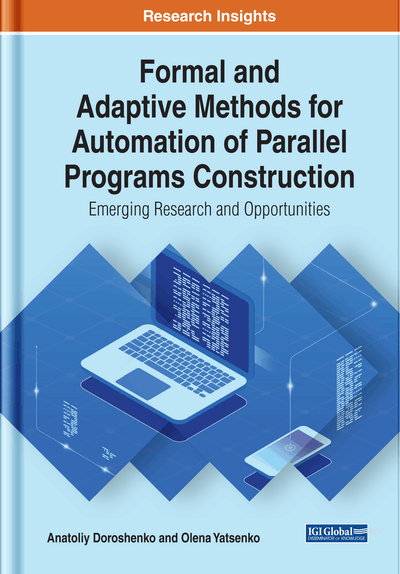# Formalized Algorithm Design and Auto-Tuning of Programs

DOI: 10.4018/978-1-5225-9384-3.ch002
Available
\$37.50
No Current Special Offers

## Abstract

This chapter deals with the process of formalized design of sequential and parallel algorithms based on algorithm algebras. It gives the main concepts associated with metarules of schemes design (convolution, involution, reinterpretation, transformation). The SAA/1 language focused on natural linguistic algorithm representation and based on Glushkov's algebras is described. The algebra-grammatical models for parameter-driven generation of algorithm specifications based on higher-level schemes (hyperschemes) are then constructed. The authors propose the extension of the well-known PRAM model that is the basis of program auto-tuning. The hyperschemes and the auto-tuning are the means of increasing the adaptability of algorithms and programs to specific conditions of their use (for example, target computing platform). Some examples of formalized design of parallel sorting algorithm schemes using operations of Glushkov's algebras are given.
Chapter Preview
Top

## Introduction

This chapter is devoted to facilities of formalized description and transformation of structured schemes of algorithms (sequential and parallel), represented in analytical and natural linguistic forms. The mentioned facilities are based on V. M. Glushkov’s system of algorithmic algebras considered in Chapter 1. The following metarules of schemes design are examined: convolution (abstracting), involution (detailed elaboration), reorientation (combination of convolution and involution) and transformation (modification of a scheme by means of application of equalities). Metarules are applied for transition between algorithms and generation of new algorithmic knowledge. The algorithmic language SAA/1 considered in this chapter is intended for multilevel structured designing and documenting of algorithms and programs in a natural linguistic form.

One of the essential problems of the algebra of algorithmics (Andon, Doroshenko, Tseytlin, & Yatsenko, 2007) is to increase the adaptability of programs to specific conditions of their use (e.g., optimizing application code for a given parallel platform). In particular, the problem can be solved by parameter-driven generation of algorithm specifications based on higher-level algorithms which are called hyperschemes (Yatsenko, 2012; Yushchenko, Tseytlin, & Galushka, 1989). The hyperscheme is a parameterized algorithm for solving a certain class of problems; setting specific values of parameters and subsequent interpretation of a hyperscheme allows obtaining algorithms adapted to specific conditions of their use. Hyperschemes are adjacent to well-known methods of transformational synthesis: term rewriting systems (Baader & Nipkow, 1999; Doroshenko & Shevchenko, 2006), mixed computations (Bulyonkov, 1990) and macrogeneration (Dybvig, 2000). In this chapter, the approach to development of sequential and parallel schemes of algorithms is considered, which is based on the use of algebra-grammatical means of parameter-driven generation of algorithm specifications.

Another approach to program optimization is provided by auto-tuning (Durillo & Fahringer, 2014; Naono, Teranishi, Cavazos, & Suda, 2010; Tichy, 2014), which is the methodology automating the search for the optimal program version out of a set of provided possibilities by running each candidate and measuring its performance on a given computing architecture. In this chapter, the parallel computation model for auto-tuning based on the extension of the well-known abstract parallel machine with random memory access (PRAM) (Eppstein & Galil, 1988) is considered.

The chapter also contains the examples of formalized design of parallel sorting algorithms using the facilities of the modified system of algorithmic algebra SAA (SAA-M) considered in Chapter 1.

## Key Terms in this Chapter

SAA/1: The algorithmic language based on Glushkov’s system of algorithmic algebras and focused on natural linguistic representation of schemes. It is applied for multilevel structured designing and documenting of sequential and parallel algorithms and programs.

Parallel Random-Access Machine (PRAM): A shared-memory abstract machine for modeling parallel algorithmic performance.

Regular Scheme (RS): A representation of an algorithm in the system of algorithmic algebras (SAA).

SAA Scheme: A representation of an algorithm in algorithmic language SAA/1.

Glushkov’s System of Algorithmic Algebras (SAA, Glushkov’s Algebra): The two-sorted algebra focused on analytical form of representation of algorithms and formalized transformation of these representations.

Modified System of Algorithmic Algebras (SAA-M): The extension of the Glushkov’s system of algorithmic algebras (SAA) intended for formalization of parallel algorithms.

Hyperscheme: A parameterized algebra-algorithmic specification intended for solving a specific class of problems. By setting parameter values with subsequent interpretation of a hyperscheme, an algorithm scheme optimized to specific conditions of its use is obtained.

PRAM*: The model which extends the classic shared-memory abstract machine for modeling parallel algorithmic performance (PRAM) with additional memory level and uses only one strategy for management of simultaneous access to both memory levels.

Algebra of Hyperschemes: The formalism applied for parameter-driven generation of regular schemes of algorithms based on higher-level specifications (hyperschemes).

Transformation: The process of conversion of algorithm schemes based on equalities represented in algorithmic algebra.

## Complete Chapter List

Search this Book:
Reset